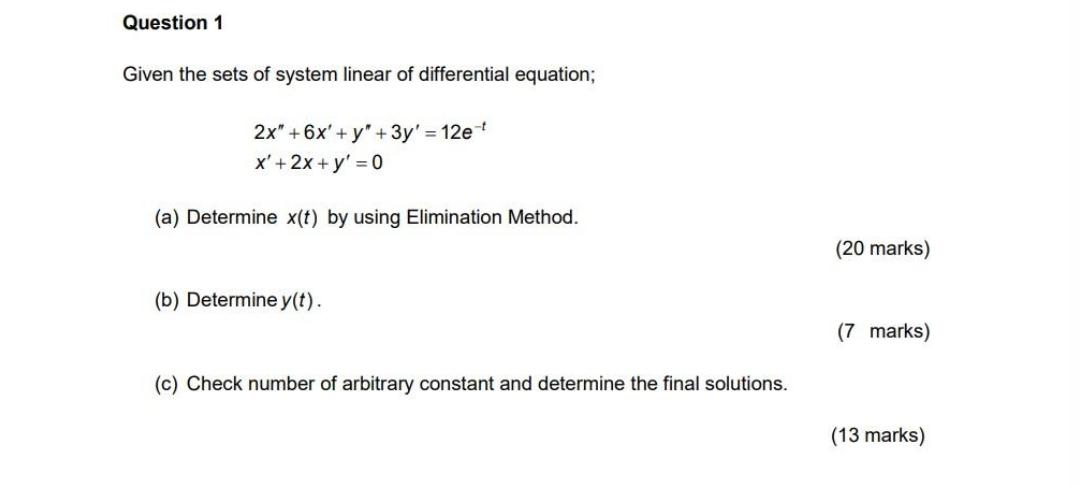Question:

# Given the sets of system linear of differential equation;

Last updated: 8/7/2022Given the sets of system linear of differential equation; 2x"+6x'+y" + 3y' = 12e ^-t x'+2x + y' = 0 (a) Determine x(t) by using Elimination Method. (b) Determine y(t). (c) Check number of arbitrary constant and determine the final solutions.